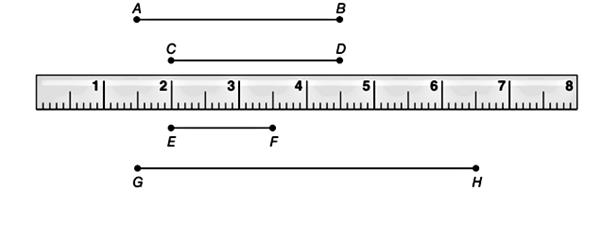Chapter P.3, Problem 18EElementary Geometry For College St...

7th Edition
Alexander + 2 others
ISBN: 9781337614085

Solutions

Chapter
SectionElementary Geometry For College St...

7th Edition
Alexander + 2 others
ISBN: 9781337614085
Textbook Problem

Judging from the ruler, estimate the measure of each line segment. a) E F b) G HTo determine

a) To estimate:

The measure of the line segment EF.

Explanation

Calculation:

Given,

Here, the point E of the line segment is at 2 cm and the point F is at 3.5 cm, thus the length of EF¯ is given by

EF=3

To determine

b) To estimate:

The measure of the line segment GH.

Still sussing out bartleby?

Check out a sample textbook solution.

See a sample solution

The Solution to Your Study Problems

Bartleby provides explanations to thousands of textbook problems written by our experts, many with advanced degrees!

Get Started

Solve the equations in Exercises 126. x4x+1xx1=0

Finite Mathematics and Applied Calculus (MindTap Course List)

Write the following numbers in word form. 3. .081

Contemporary Mathematics for Business & Consumers

The equation of the line through (4, 10, 8) and (3, 5, 1) is:

Study Guide for Stewart's Multivariable Calculus, 8th